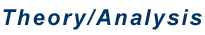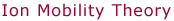Kinetics - Direct Measurement     In the direct-measurement method for investigating kinetics, ions are injected into the drift cell where reactions takes place. For fragmentation reactions, A+B+ + C, the drift cell contains an unreactive buffer gas and the fragmentation kinetics are governed by the first order rate law.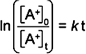In this expression, [A+]0 is the initial concentration of the reactant ion A+ and [A+]t is the concentration of A+ after a reaction time of t. The reaction time is equal to the time the ions spend in the cell, i.e. the drift time. The drift time, td, can be varied by changing the cell drift voltage or the pressure of the gas in the cell and is determined by the method described in the Ion Mobility Theory Introduction. The concentration ratio is obtained from the mass spectrum of the ions measured after they exit the drift cell. For the general reaction A+B+ + C, the initial concentration of A+ is equal to the sum of the concentrations of A+ and B+ at time t. The concentrations are directly proportional to the intensities of the peaks in the mass spectrum.*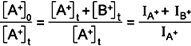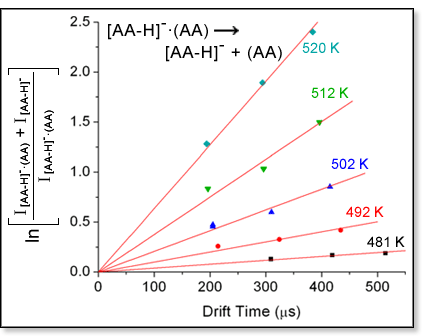In this expression, IA+ and IB+ are the intensities of the A+ and B+ peaks in the mass spectrum, respectively. A plot of the natural log of the peak intensity ratio as a function of reaction time yields a straight line with a slope equal to the rate constant k. The figure at left shows an example of this type of plot at several temperatures for the dissociation of the dialanine dimer [Liu, D.; Wyttenbach, T.; Carpenter, C. J.; Bowers, M. T. J. Am. Chem. Soc. 2004, 126, 3261-3270]. By obtaining rate constants over a range of temperatures, an Arrhenius analysis of the reaction can be carried out. The Arrhenius equation indicates that plotting ln k as a function of 1/T will yield a straight line with a slope proportional to the activation energy Ea.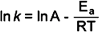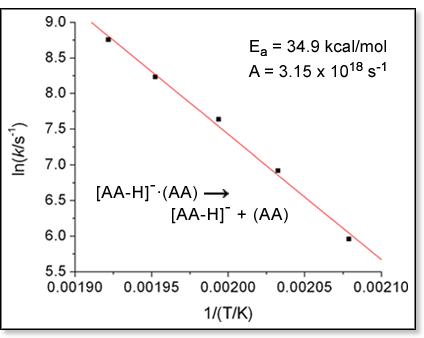The preexponential factor A, which is related to the entropy of activation for the reaction, can be obtained from the y-intercept of the line. An example of an Arrhenius plot is shown at right for the peptide dimer dissociation reaction.     The direct-measurement kinetics method has also been used to determine rate constants for bimolecular reactions with multiple product channels, A+ + Bproductsi [Manard, M. J.; Kemper, P. R.; Carpenter, C. J.; Bowers, M. T. Int. J. Mass Spectrom. 2005, 241, 99-108]. For these types of reactions, ions are injected into the drift cell containing the neutral reactant. The reactions can be considered pseudo-first order under our experimental conditions since the neutral reactant B is present in much greater concentrations in the drift cell than the ionic reactant A+. In these cases the plot generated to determine the rate constants will have a slope that incorporates the number density of the neutral reactant gas ρ(B).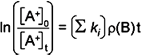In this expression, Σki is the sum of the rate constants for each product channel.   * Note: This assumes instrumentational mass discrimination is insignificant. Experience has shown this to be the case in all of the systems we have studied.